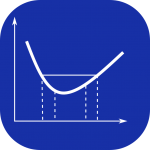# Convexity of object edges with image processingThe local convexity of object contours can be determined and used for further geometric analysis. A mathematical curve is convex if its second derivative is greater than 0 at each point. It can also be said that the function rotates counterclockwise. A convex function is therefore ‘open to the top’ and looks contrary to the physical convex surface.

Convexity in image processing indicates how far the contour of an object deviates from a convex outline. This can then be used to detect incisions or constrictions.

This measurement parameter is e.g. used for the quantification of round objects such as Lemna-Fronds or the counting of individual cells in cell aggregates (conglomerate separation).